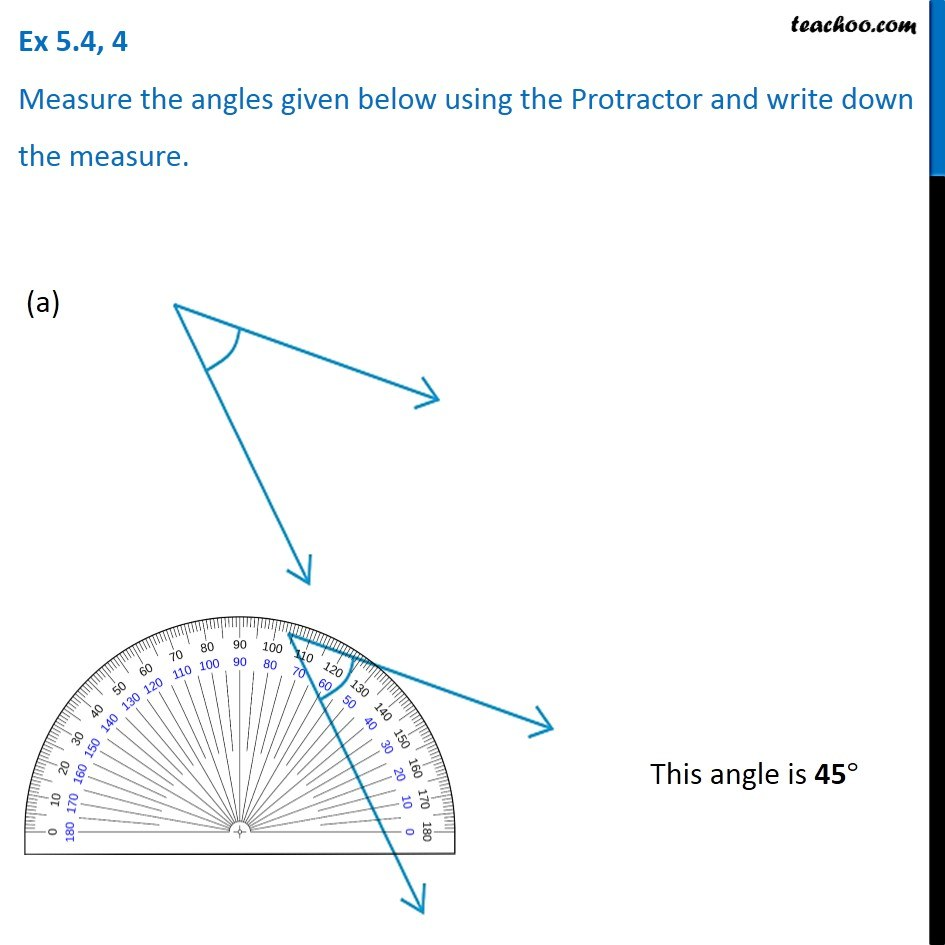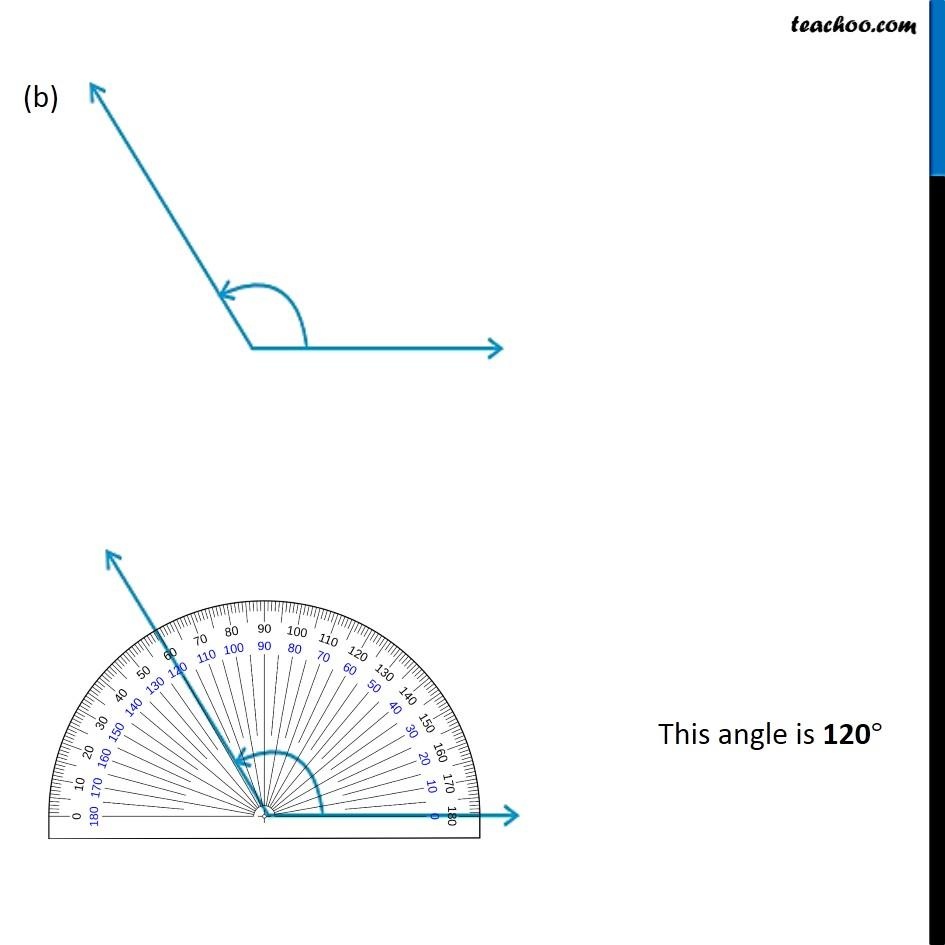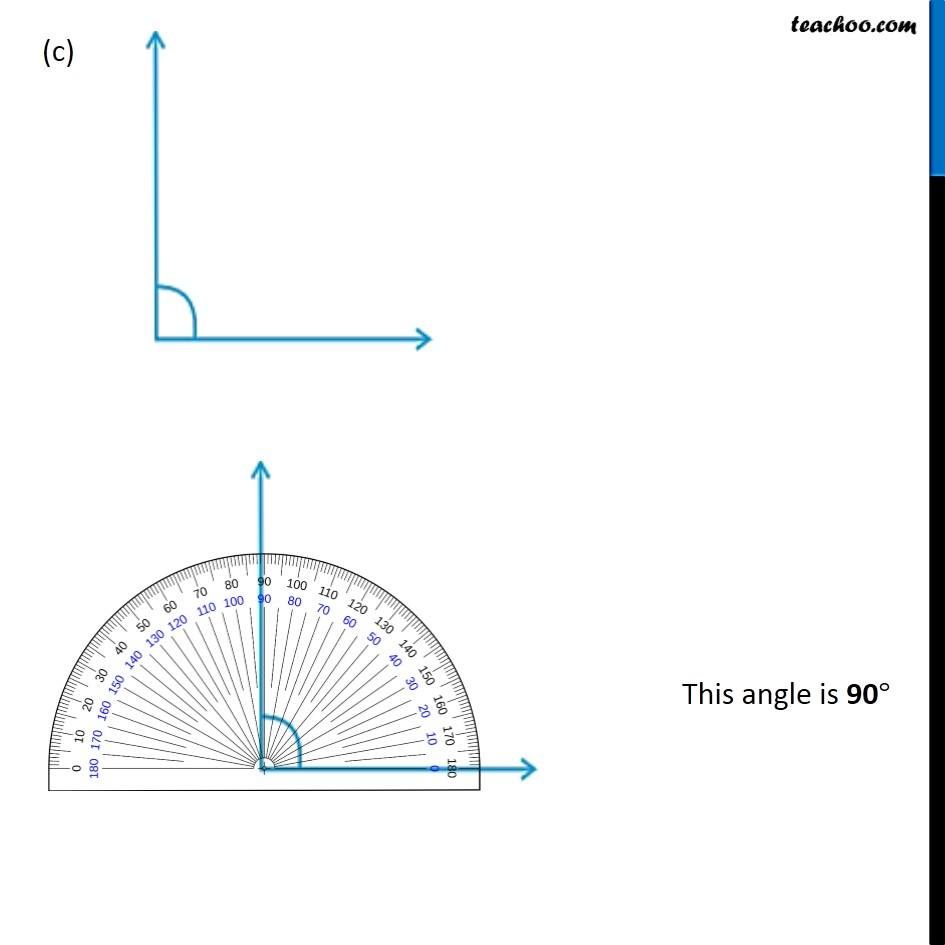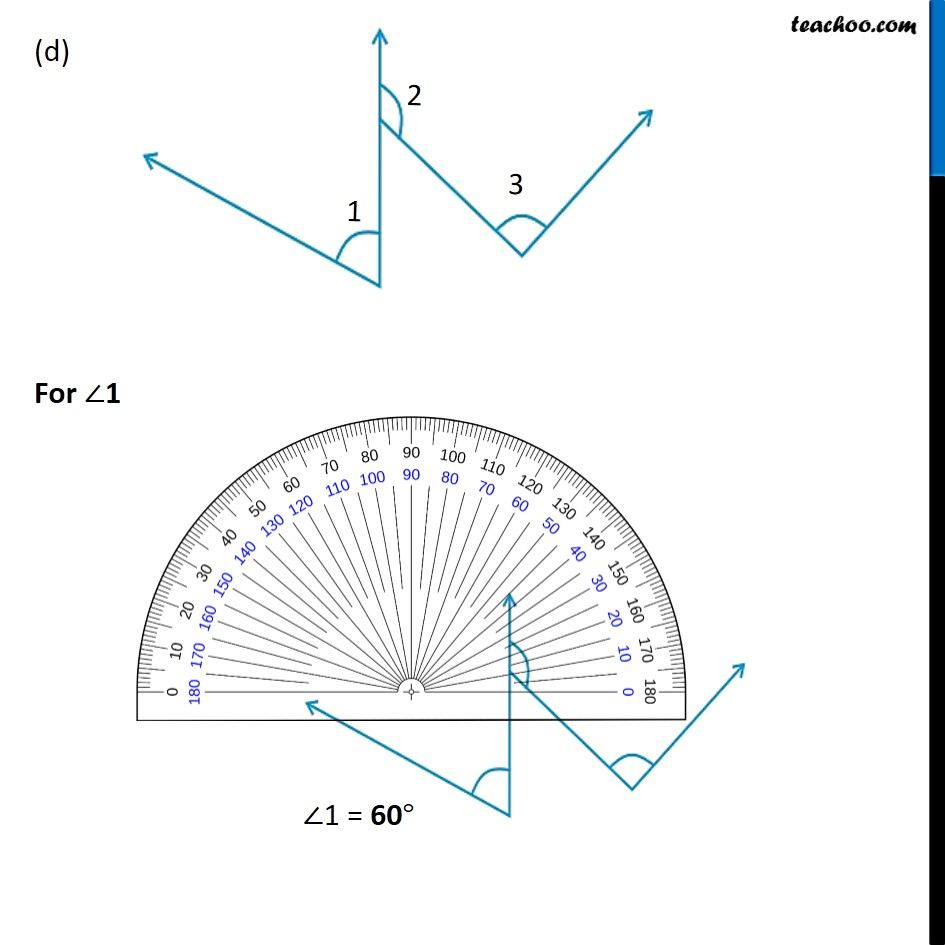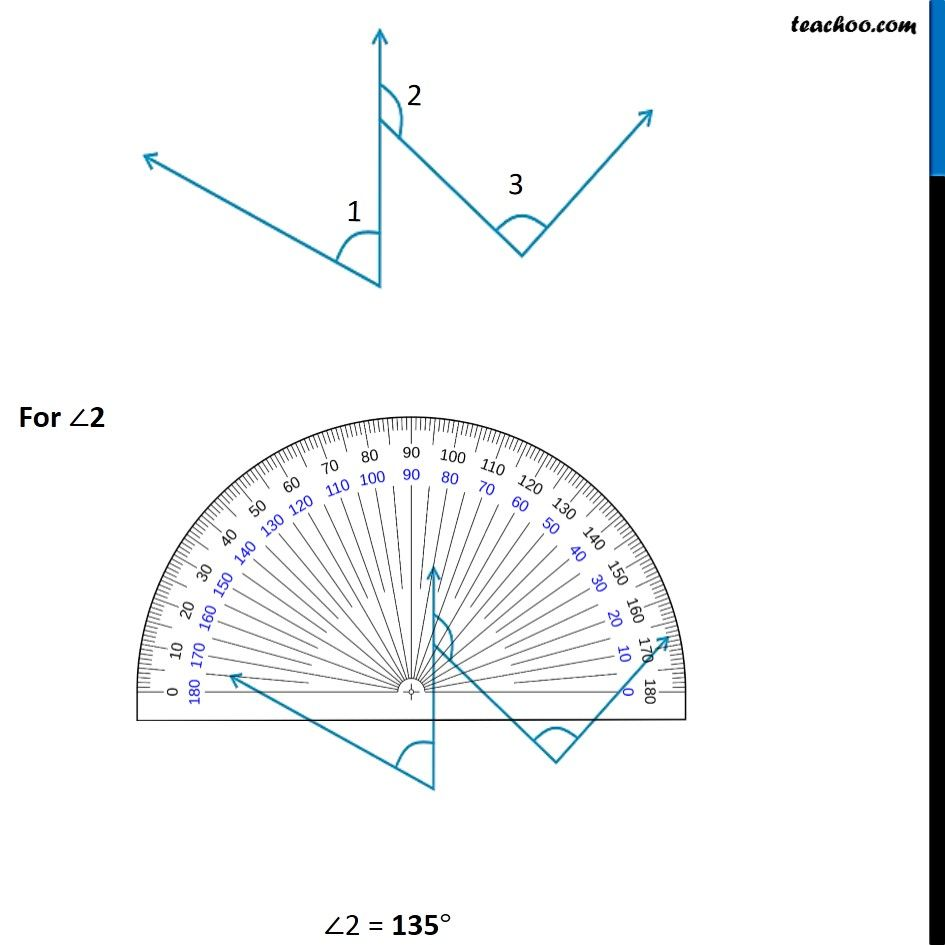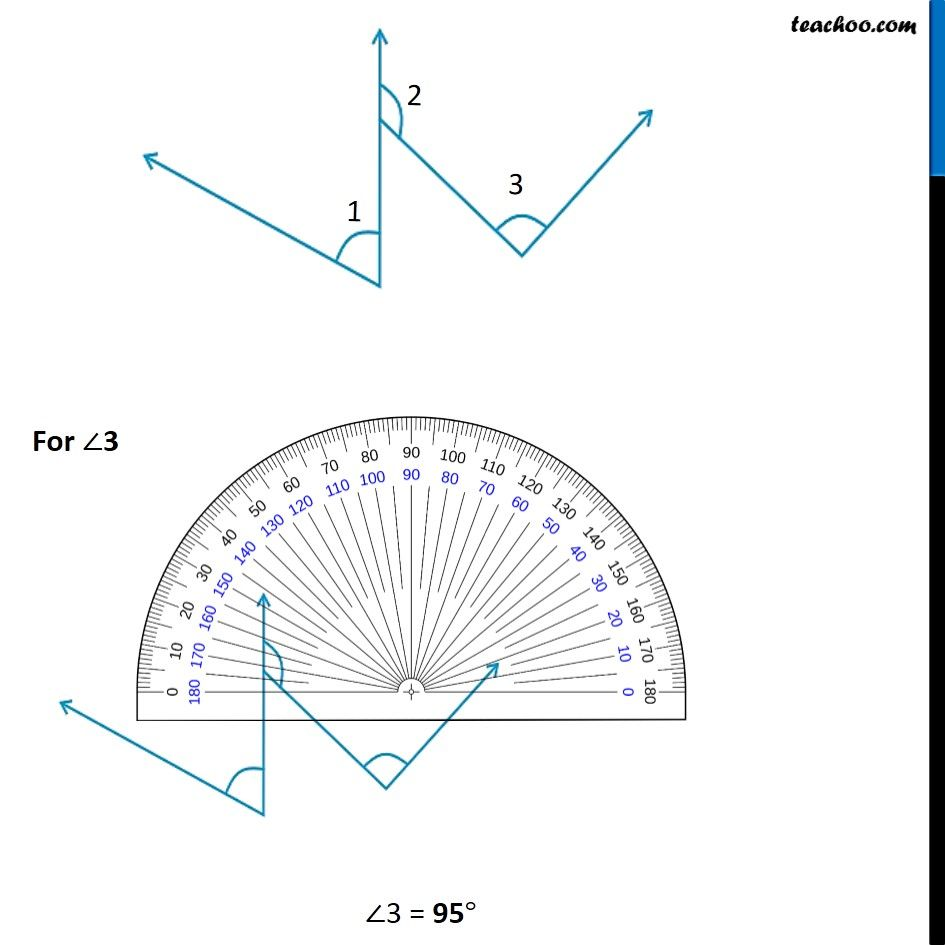Subscribe to our Youtube Channel - https://you.tube/teachoo

1. Chapter 5 Class 6 Understanding Elementary Shapes
2. Concept wise
3. Measuring angles using protractor

Transcript

Ex 5.4, 4 - Chapter 5 Class 6 Understanding Elementary Shapes Measure the angles given below using the Protractor and write down the measure. (a) This angle is 45° (b) This angle is 120° (c) This angle is 90° (d) For ∠1 ∠1 = 60° For ∠2 ∠2 = 135° For ∠3 ∠3 = 95°

Measuring angles using protractor